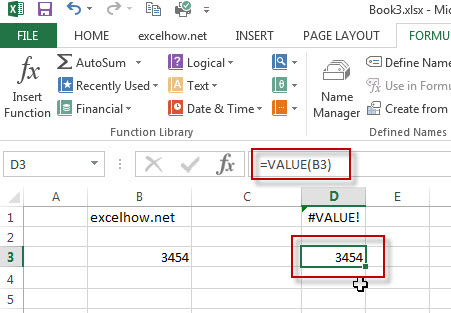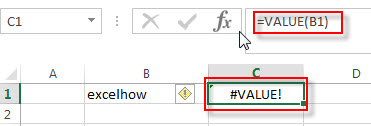# Excel Value Function

This post will guide you how to use Excel VALUE function with syntax and examples in Microsoft excel.

## Description

The Excel VALUE function converts a text string that represents a number to a numeric value.

The VALUE function is a build-in function in Microsoft Excel and it is categorized as a Text Function.

The VALUE function is available in Excel 2016, Excel 2013, Excel 2010, Excel 2007, Excel 2003, Excel XP, Excel 2000, Excel 2011 for Mac.

## Syntax

The syntax of the VALUE function is as below:

`= VALUE (text)`

Where the VALUE function argument is:

• Text-This is a required argument. The text string that you want to convert it to a number.

Note:

• Text can be in any of the constant number, date, or time formats. And if text is not a number, the VALUE function will return #VALUE! Error.
• Normally, you do not need to use the VALUE function in a formula, because Excel converts text string to numeric value as necessary automatically. It is provided for compatibility with other spreadsheet programs.

## Excel VALUE Function Example

The below examples will show you how to use Excel VALUE function to convert string to a number.

#1 To convert a text in B3 cell to a number, just using formula:

`= VALUE(B3)`#2 to convert a text string that is not a recognized format (number, date, time format) into a numeric value, using the below formula:

`=VALUE(B1)`You will see that the #VALUE! Error will be returned by the VALUE function. Because the text is not a number.

### More Excel VALUE Formula Examples

• Convet Text Date dd/mm/yy to mm/dd/yyyy as Date Format
If you want to convert dates in the text format to the date format as mm/dd/yyyy, you can use a combination of the DATE function, the VALUE function, the RIGHT function, the MID function, and the LEFT function to create a new excel formula…
• Remove Apostrophe or Text Indicator
If you want to remove the text indicator (‘) or the leading Apostrophe from a text string in one cell, you can create a formula based on the VALUE function….